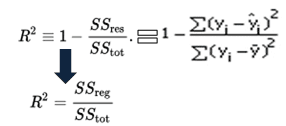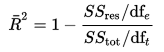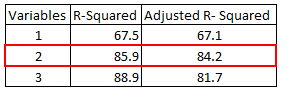# Difference between Adjusted R-squared and R-squared

In this tutorial, we will cover the difference between r-squared and adjusted r-squared. It includes detailed theoretical and practical explanation of these two statistical metrics in R.

## R-squared (R²)

It measures the proportion of the variation in your dependent variable explained by all of your independent variables in the model. It assumes that every independent variable in the model helps to explain variation in the dependent variable. In reality, some independent variables (predictors) don't help to explain dependent (target) variable. In other words, some variables do not contribute in predicting target variable.

Mathematically, R-squared is calculated by dividing sum of squares of residuals (SSres) by total sum of squares (SStot) and then subtract it from 1. In this case, SStot measures total variation. SSreg measures explained variation and SSres measures unexplained variation.

As SSres + SSreg = SStot, R² = Explained variation / Total VariationR-squared Equation
R-Squared is also called coefficient of determination. It lies between 0% and 100%. A r-squared value of 100% means the model explains all the variation of the target variable. And a value of 0% measures zero predictive power of the model. Higher R-squared value, better the model.

It measures the proportion of variation explained by only those independent variables that really help in explaining the dependent variable. It penalizes you for adding independent variables that do not help in predicting the dependent variable in regression analysis.

Adjusted R-Squared can be calculated mathematically in terms of sum of squares. The only difference between R-square and Adjusted R-square equation is degree of freedom.Adjusted R-Squared Equation
In the above equation, dft is the degrees of freedom n– 1 of the estimate of the population variance of the dependent variable, and dfe is the degrees of freedom n – p – 1 of the estimate of the underlying population error variance.

Adjusted R-squared value can be calculated based on value of r-squared, number of independent variables (predictors), total sample size.Adjusted R-Squared Equation 2

## Difference between R-square and Adjusted R-square

1. Every time you add a independent variable to a model, the R-squared increases, even if the independent variable is insignificant. It never declines. Whereas Adjusted R-squared increases only when independent variable is significant and affects dependent variable.

2. In the table below, adjusted r-squared is maximum when we included two variables. It declines when third variable is added. Whereas r-squared increases when we included third variable. It means third variable is insignificant to the model.R-Squared vs. Adjusted R-Squared
3. Adjusted r-squared can be negative when r-squared is close to zero.
4. Adjusted r-squared value always be less than or equal to r-squared value.
Which is better?
Adjusted R-square should be used to compare models with different numbers of independent variables. Adjusted R-square should be used while selecting important predictors (independent variables) for the regression model.

## Understand Adjusted R-squared with Example

Suppose you want to find out the relationship between the impact of advertising expenditure (X1) and the price of the product (X2) on the sales (Y).

Sales = β0 + β1 * Advertising + β2 * Price

Here β0 is intercept, β1 refers to slope/coefficient for advertising expenditure and β2 is coefficient/slope for the price of the product.

Let's say you run the linear regression model and the R-squared value came out 0.8 which means that 80% of the variation in sales can be explained by the advertising expenditure and the price of the product. Whereas the adjusted R-squared value would be lower than the R-squared value.

## R : Calculate R-Squared and Adjusted R-Squared

Suppose you have actual and predicted dependent variable values. In the script below, we have created a sample of these values. In this example, y refers to the observed dependent variable and yhat refers to the predicted dependent variable.
```y = c(21, 21, 22.8, 21.4, 18.7, 18.1, 14.3, 24.4, 22.8, 19.2)
yhat = c(21.5, 21.14, 26.1, 20.2, 17.5, 19.7, 14.9, 22.5, 25.1, 18)
R.squared = 1 - sum((y-yhat)^2)/sum((y-mean(y))^2)
print(R.squared)
```
Final Result : R-Squared = 0.6410828

Let's assume you have three independent variables in this case.
```n = 10
p = 3
adj.r.squared = 1 - (1 - R.squared) * ((n - 1)/(n-p-1))
```
In this case, adjusted r-squared value is 0.4616242 assuming we have 3 predictors and 10 observations.

## Python : Calculate Adjusted R-Squared and R-Squared

```import numpy as np
y = np.array([21, 21, 22.8, 21.4, 18.7, 18.1, 14.3, 24.4, 22.8, 19.2])
yhat = np.array([21.5, 21.14, 26.1, 20.2, 17.5, 19.7, 14.9, 22.5, 25.1, 18])
R2 = 1 - np.sum((yhat - y)**2) / np.sum((y - np.mean(y))**2)
R2
n=y.shape
p=3
adj_rsquared = 1 - (1 - R2) * ((n - 1)/(n-p-1))
```

In the code above, we are calculating R-squared value using the formula: `1 - (sum of squared residuals / sum of squared total variation)`. The squared residuals are obtained by subtracting the predicted values (yhat) from the observed values (y), then we square the differences, and sum them up. The squared total variation is obtained by subtracting the mean of the observed values (np.mean(y)) from each observed value, then we square the differences, and sum them up. Finally, the R-squared value is calculated by subtracting the ratio of squared residuals to squared total variation from 1

Adjusted R-squared value is calculated using the formula: 1 - (1 - R-squared) * ((n - 1)/(n - p - 1)). Here, n represents the number of observations, and p represents the number of predictors (independent variables) in the regression model.

## SAS : Adjusted R-Squared Calculation

```data temp;
input y yhat;
cards;
21 21.5
21 21.14
22.8 26.1
21.4 20.2
18.7 17.5
18.1 19.7
14.3 14.9
24.4 22.5
22.8 25.1
19.2 18
;
run;

data out2;
set temp ;
d=y-yhat;
absd=abs(d);
d2 = d**2;
run;

/* Residual Sum of Square */
proc means data = out2 ;
var d2;
run;

data _null_;
run;

/* Total Sum of Square */
proc means data = temp ;
var y;
output out=avg_y mean=avg_y;
run;

data _null_;
set avg_y;
call symputx ('avgy', avg_y);
run;

%put &avgy.;

data out22;
set temp ;
diff = y - &avgy.;
diff2= diff**2;
run;

proc means data = out22 ;
var diff2;
output out=TSS sum=;
run;

data _null_;
set TSS;
call symputx ('TSS', diff2);
run;

/* Calculate the R2 */
%put &RSQ;

/* Calculate the Adj R2 */
%LET N = 10;
%LET P = 3;
```

We have created a new dataset named `out2` from the `temp` dataset. It calculates the differences between the actual (y) and predicted (yhat) values and stores them in the variable d. The absolute differences are stored in the variable absd. The squared differences are stored in the variable d2. `proc means` procedure was used to calculate the sum of squared differences ("d2") in the "out2" dataset.

The proc means procedure is used again to calculate the mean of y and store it in the variable avg_y. Then the differences between each actual value and the mean are calculated and squared, resulting in the variable diff2. Finally, we used the proc means procedure one more time to calculate the sum of squared differences diff2 in the out22 dataset, and the result (TSS) is stored in the dataset named TSS.Share
Related PostsDeepanshu founded ListenData with a simple objective - Make analytics easy to understand and follow. He has over 10 years of experience in data science. During his tenure, he has worked with global clients in various domains like Banking, Insurance, Private Equity, Telecom and Human Resource.

9 Responses to "Difference between Adjusted R-squared and R-squared"
1.Could you please explain RMSE, AIC and BIC as well.
We use RMSE to compare our model. Lesser the value is good for our model, but I m not sure about the rest of the statistics AIC and BIC respectively..

2.Nicely Explained

3.nice explaination so it means always adjusted r square will <= Rsquare

4.Could you give please the data set in order to understand the difference better.

5.R squared depends on the sum of squared errors (SSE), il SSE decreases (new predictor has improved the fit) then R squared increases. In this case R squared is a good measure. Please give us a complete example to understand. Thank you

6.its really good effort to explain it.. Also explain BIC and AIC

7.nicely explained

8.Good explanation

9.Next → ← Prev
Looks like you are using an ad blocker!

To continue reading you need to turnoff adblocker and refresh the page. We rely on advertising to help fund our site. Please whitelist us if you enjoy our content.